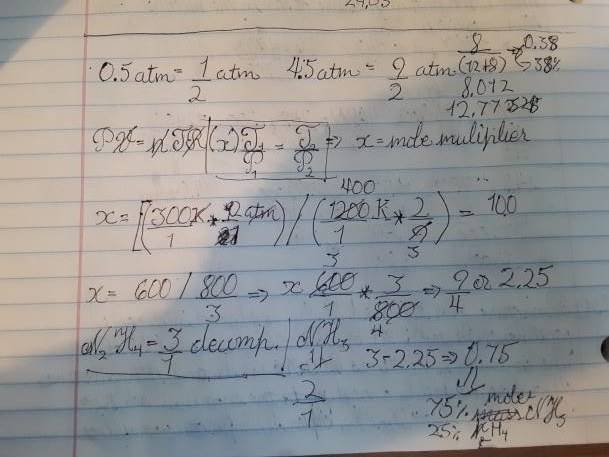# Help with Gas law problems

• Chemistry
Unart
Homework Statement:
131.) A mixture of NH3( g) and N2H4( g) is placed in a sealed container at 300 K. The total pressure is 0.50 atm. The container is heated to 1200 K at which time both substances decompose completely according to the equations 2 NH3( g) = N2( g) + 3 H2( g); N2H4( g) = N2( g) + 2 H2( g). After decomposition is complete, the total pressure at 1200 K is found to be 4.5 atm. Find the percent of N2H4( g) in the original mixture. (Assume two significant figures for the temperature.)
Relevant Equations:
PV=nRT
Pressure = P
Volume = V
moles = n
gas constant = r
temperature (kelvins) = T
So essentially you are supposed to find the mass percentage of N2H4 in the Hydrogen/Nitrogen mixtures. The way I understood it, the only way to find the mass percentage of the gasses is to find the mole percentage. Which I got as 25%, based on the fact that the ratio of product gasses to reactants is 9/4 where N2H4 is 3/1 and NH3 is 2.

I got a mass percentage of 38% ~ book says 25%